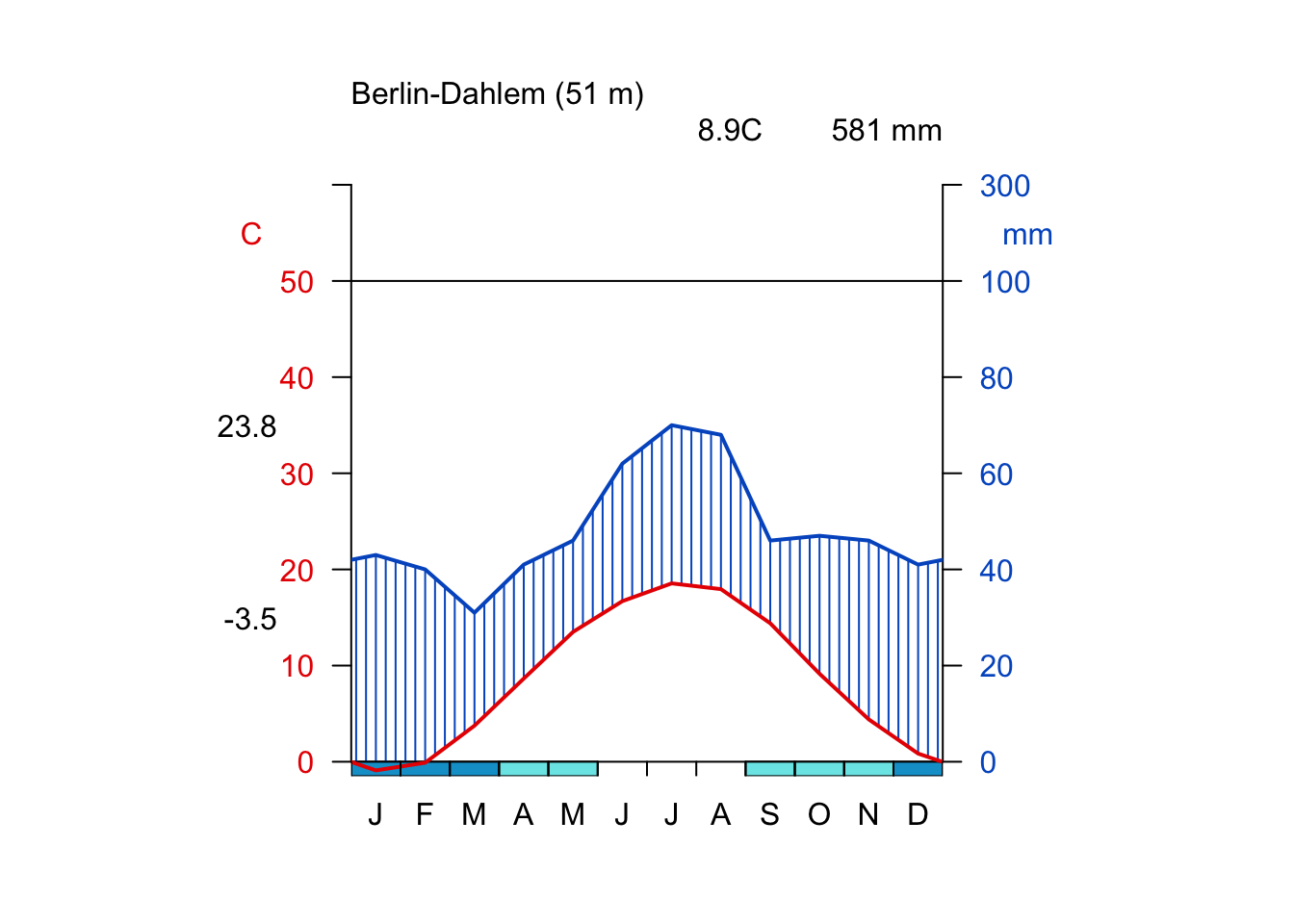A very popular form of visualizing meteorological data is the so-called Walter-Lieth graph. Such a graph illustrates precipitation and temperature changes throughout the year. It is especially useful to determine arid and humid months throughout the annual cycle.

Drawing such a graph by hand is tedious, therefore we apply the diagwl() function from the climatol package.

library(climatol)

If you get an error try to install the package by typing install.packages("climatol") into your R console.

Let us inspect the arguments of the diagwl() function using the args() command and ask for help by typing help(diagwl) into the R console.

args(diagwl)
## function (dat, est = "", alt = NA, per = "", margen = c(4, 4,
##     5, 4), mlab = "", pcol = "#005ac8", tcol = "#e81800", pfcol = "#79e6e8",
##     sfcol = "#09a0d1", shem = FALSE, p3line = FALSE, ...)
## NULL

From the help page we know that the climatic data must be passed as a 4x12 matrix of monthly (January to December) data, in the following order:

• Row 1: Mean precipitation
• Row 2: Mean maximum daily temperature
• Row 3: Mean minimum daily temperature
• Row 4: Absolute monthly minimum temperature

This last row is only used to determine the probable frost months (when absolute monthly minimums are equal or lower than 0°C). For stations located in the southern hemisphere it is useful to set shem = TRUE, in order to keep the summer period in the central zone of the graph (the diagram will begin the plot with the July data).

As described by Walter and Lieth (Klimadiagramm-Weltatlas, 1967), when monthly precipitation is greater than 100 mm, the scale is increased from 2mm/ºC to 20mm/ºC to avoid too high diagrams in very wet locations. This change is indicated by a black horizontal line, and the graph over it is filled in solid blue. When the precipitation graph lies under the temperature graph $$(P < 2T)$$ we have an arid period (filled in dotted red vertical lines). Otherwise the period is considered wet (filled in blue lines), unless p3line = TRUE, that draws a precipitation black line with a scale $$P = 3T$$; in this case the period in which $$3T > P > 2T$$ is considered semi-arid. Daily maximum average temperature of the hottest month and daily minimum average temperature of the coldest month are frequently used in vegetation studies, and are labeled in black at the left margin of the diagram.

In the example we construct a Walter-Lieth diagram for the weather station Berlin-Dahlem (Source: Müller, M.J. (1996): Handbuch ausgewählter Klimastationen der Erde)

### Data from January to December (Müller, M.J. (1996))

## Mean precipitation in mm
mean.prec <- c(43, 40, 31, 41, 46, 62, 70, 68, 46, 47, 46, 41)
## Mean maximum daily temperature
m.max.dt <- c(1.7, 2.9, 7.8, 13.5, 19.1, 22.3, 23.8, 23.3, 19.5, 13.0, 6.9, 3.1)
## Mean minimum daily temperature
m.min.dt <- c(-3.5, -3.1, -0.3, 3.8, 7.9, 11.1, 13.3, 12.6, 9.3, 5.3, 1.9, -1.4)
## Absolute monthly minimum temperature
abs.m.min.t <- c(-21, -26, -16.5, -6.7, -2.9, 1.4, 5.7, 4.7, -0.5, -9.6, -13.5, -20.2)

data.matrix <- rbind(mean.prec,
m.max.dt,
m.min.dt,
abs.m.min.t)
## altidude
alt <- 51 # m asl

diagwl(data.matrix,
est = "Berlin-Dahlem",
alt = alt,
#per = "1961-90",
mlab = "en")